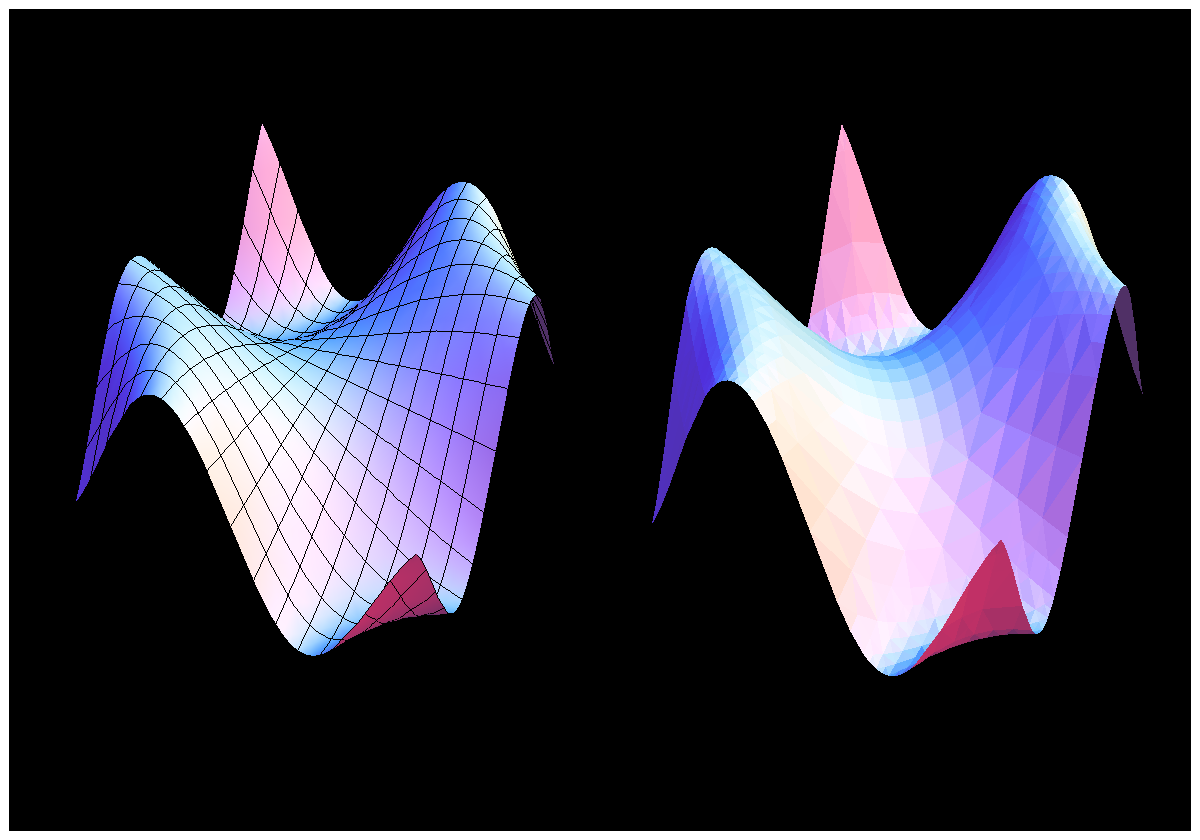# Code

#### The drinkable proof

archimedes.m (TXT file for Mathematica)
```(* Drinkable proof of the Archimedes idea to relate the volume of the sphere with the complement of the volume of a cone in a cylinder   *)
(* Oliver Knill and Elizabeth Slavkovsky. This Mathematica file was written on December 11, 2011 and put online on February 3, 2013      *)
(* See http://arxiv.org/abs/1301.5027 for the paper and http://www.math.harvard.edu/~knill/3dprinter for the project page                *)

M=6; a=0.1; b=0.06; b1=Sin[b]; b2=Cos[b]; b1a=ArcSin[b1/(1+a)]; b2a=(1+a)Cos[b1a]; opacity=1;  o=opacity;
bowl1   =ParametricPlot3D[{Cos[t] Sin[s],Sin[t] Sin[s],Cos[s]}+{0,0,2},{t,0,2Pi},{s,Pi/2,Pi-b},Mesh->False,PlotStyle->{Yellow,Opacity[o]}];
bowl2   =ParametricPlot3D[(1+a){Cos[t] Sin[s],Sin[t] Sin[s],Cos[s]}+{0,0,2},{t,0,2Pi},{s,Pi/2,Pi-b1a},Mesh->False,PlotStyle->{Yellow,Opacity[o]}];
drain   =ParametricPlot3D[{b1 Cos[t],b1 Sin[t],z},{z,2-b2,2-b2a},{t,0,2Pi},Mesh->False,PlotStyle->{Red,Opacity[o]}];
cone1   =ParametricPlot3D[{(1-z)Cos[t],(1-z)Sin[t],z-1},{t,0,2Pi},{z,0,1},Mesh->False,PlotStyle->{Green,Opacity[o]}];
cone2   =ParametricPlot3D[{(1+a)(1-z) Cos[t],(1+a)(1-z) Sin[t],z-1},{t,0,2Pi},{z,0,1}, Mesh->False,PlotStyle->{Green,Opacity[o]}];
wall1   =ParametricPlot3D[{Cos[t],Sin[t],z},{t,0,2Pi},{z,-1,0},PlotStyle->{Blue,Opacity[o]},Mesh->False];
wall2   =ParametricPlot3D[{(1+a)Cos[t],(1+a)Sin[t],z},{t,0,2Pi},{z,-1,0},PlotStyle->{Blue,Opacity[o]},Mesh->False];
top1    =ParametricPlot3D[{r Cos[t],r Sin[t],0},{r,1,1+a},{t,0,2Pi},Mesh->False,PlotStyle->{Red,Opacity[o]}];
top2    =ParametricPlot3D[{r Cos[t],r Sin[t],2},{r,1,1+a},{t,0,2Pi},Mesh->False,PlotStyle->{Red,Opacity[o]}];
connect =Graphics3D[{Opacity[o],Table[m=2Pi*k/M;a2=a/2;{Blue,Cylinder[{{(1+a2)Cos[m],(1+a2)Sin[m],-1},{(1+a2)Cos[m],(1+a2)Sin[m],2}},a2]},{k,M}]}];
bottom  =ParametricPlot3D[{r Cos[t],r Sin[t],-1},{r,0, 1+a},{t,0,2Pi},Mesh->False,PlotStyle->{Blue,Opacity[o]}];

S=Show[{connect,drain,bowl1,bowl2,cone1,cone2,wall1,wall2,bottom,top1,top2},PlotRange->All,Boxed->False,Axes->False]

Export["archimedes.3ds",S,"3DS"]    (* to import into other graphics programs like sketchup to place into google earth and fly through  *)
Export["archimedes.wrl",S]          (* to print in color. Color printing services often can not print this in color yet                 *)
Export["archimedes.stl",S]          (* the standard STL export which can be printed on 3D printers                                      *)
```

#### Reading and Writing STL files with Mathematica

 ```S=Plot3D[Sin[x y],{x,-2,2},{y,-2,2}]; Export["test.stl",S,"STL"]; A=Import["test.stl"]; Show[A] ``` ```A graphic object in Mathematica. Here a graph. Export the STL file Import an STL file Show the STL file ```

#### Writing WRL files with Mathematica

See Mathematica project in Math21a of Fall 2012:
 ```S=SphericalPlot3D[(2+Sin[8 t+s] Sin[5 s]),{t,0,Pi},{s,0,2 Pi}] Export["test.wrl",S]; ``` ```A graphic object in Mathematica. Export the WRL file ```
Remarks:
1. Variants like Export["test.wrl",S,"WRL], or Export["test.wrl",S,"WVRL"] does not work (at least not in Mathematica 8).
2. Even if the WRL file can be exported, don't expect it to be printable in color. Sometimes it does, sometimes not.
3. WRL files can currently not yet be imported in Mathematica. Meshlab can import it but the color is lost.To the left the Mathematica graphics object. Right after exporting and importing again. We see the triangularization.

#### Producing ASCII STl files

```admesh -a out.stl in.stl
```
converts a binary stl file into an ascii stl file. By default, this is not reversible.
```admesh -b in.stl out.stl
```